## Band Stop Filter:

Band Stop Filter stop a range of frequencies between two cut-off frequencies f1 and f2 while pass all the frequencies below f1 and above f2. Thus range of frequencies between f1 and f2 constitutes a stop band in which attenuation to the frequencies is infinite ideally. The frequencies below f1 and above f2 constitute two separate pass bands in which attenuation to the frequencies is zero ideally.

The Band Stop Filter can be obtained by connecting low pass filter and high pass filter sections in parallel where cut-off frequency of the low pass filter section is less than that of the high pass filter section. But the economial form of the band elimination filter can be obtained by combining the low pass and high pass filter section if series, arm contains parallel resonant circuit while shunt arm contains series resonant circuit as shown in the Fig. 9.20 (a) and (b).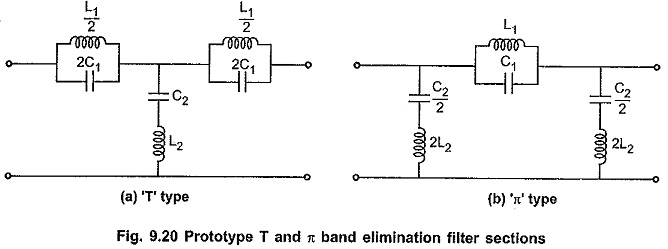The band elimination characteristics can be obtained by using conventional Band Stop Filter (either T or π type) as shown in the Fig. 9.20, if the series resonant frequency of the shunt arm is selected same as the parallel resonant frequency of the series arm. Consider ‘T’ type band elimination filter section as shown in the Fig. 9.20(a).

Let the frequency of the series and shunt arm be ω0 rad/sec. Then for series arm, frequency of anti resonance is given by,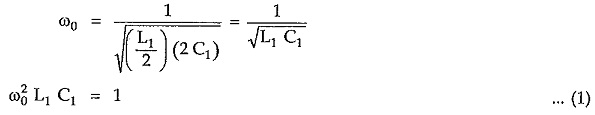Similarly, for shunt arm, frequency of resonance is given by,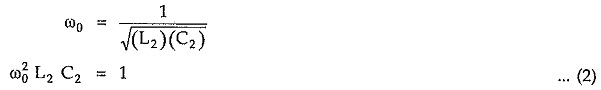From equations (1) and (2), for same resonant frequencies of series and shunt arm resonant circuit we can write,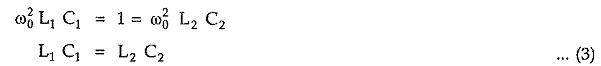### Design Impedance (R0):

Total series arm impedance is given by,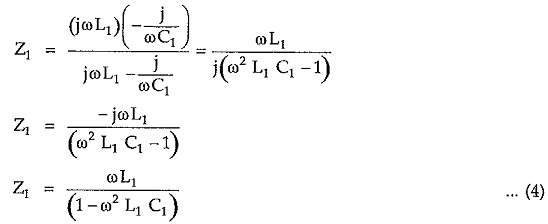Similarly, total shunt arm impedance Z2 is given by,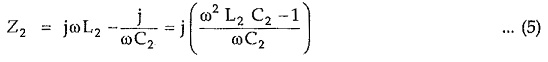Hence Z1Z2 = L2/C1 = L2/C2 which is real and constant. Hence above sections are constant k sections. So we can write,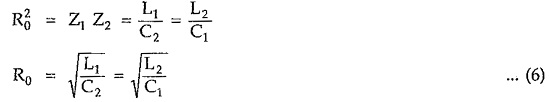#### Reactance Curves and Expressions for Cut-off Frequencies:

To verify the band elimination characteristics, let Z1 =j X1 and Z2 =j X2. The reactance curves of X1 and X1/4 + X2 against frequency are as shown in the Fig. 9.21.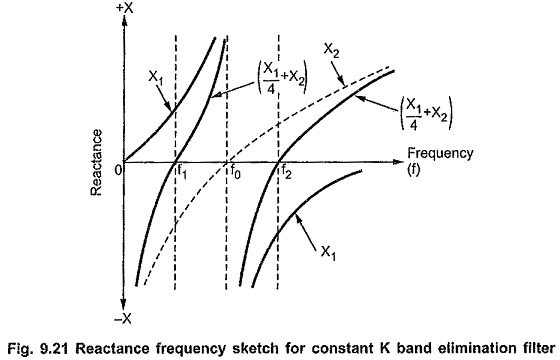From the above characteristics it is clear that the reactance curves for X1 and (X1/4 + X2) are on the same sides of the frequency axis between f1 and f2 which indicates stop band. These curves are on opposite sides of the axis below f1 and above f2 which indicates two pass band. Hence for the given section, the characteristics are of band elimination filter where f1 and f2 are the cut-off frequencies.

In Band Stop Filter, the condition for cut-off frequencies is given by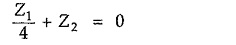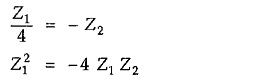But from the condition of constant K filter section, Z1Z2 = R20From above equation it is clear that the value of the series arm impedance Z1 can be obtained at two different cut-off frequencies namely f1 and f2. So at f = f1, Z1 = + j(2 R0) and at f = f2, Z= -j(2 R0). Thus impedance Z1 at f1, i.e. at lower cut-off frequency, is negative of the impedance Z1 at f2 i.e. upper cut-off frequency. Hence we can write,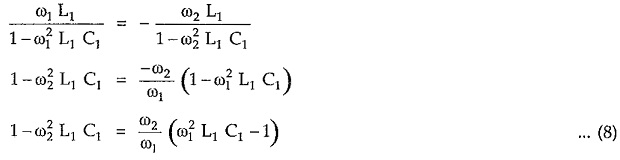But from equation (1), frequency of resonance is given by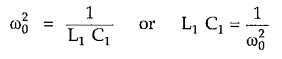Substituting value of (L1 C1 ) in above equation, we can write,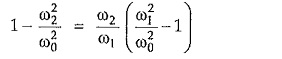Simplifying above equation,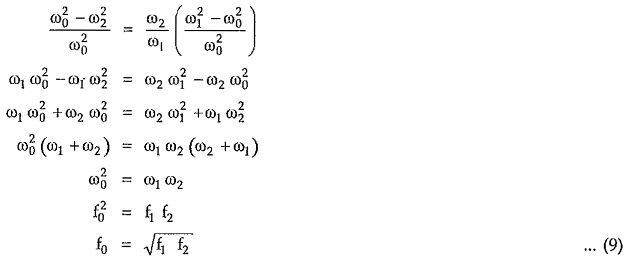Hence, above equation (9) indicates that in band elimination filter, the frequency of resonance of the individual arms is the geometric mean of two cut-off frequencies.

#### Variation of Z0T and Z0π, Attenuation Constant (α), Phase Constant (β) with Frequency:

The variations of Z0T  and Z, attenuation constant (α) and phase shift (β) are as shown in the Fig. 9.22 (a), (b) and (c) respectively. Consider that f1 and f2 are two cut-off frequencies and R0 is the design impedance of the Band Stop Filter.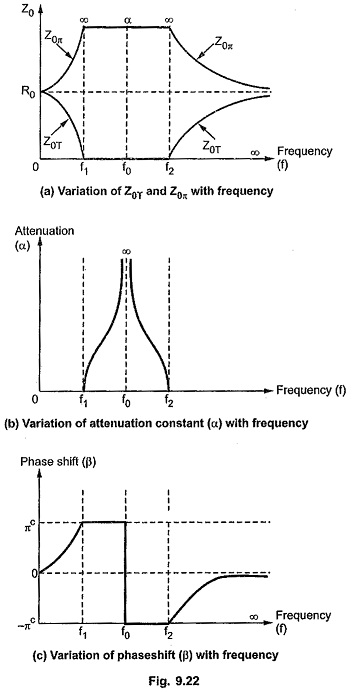#### Design Equations:

Consider that a band elimination filter with two cut-off frequencies f1 and f2 is terminated in design impedance R0. Then, from equation (7), at lower cut-off frequency f1, we can write,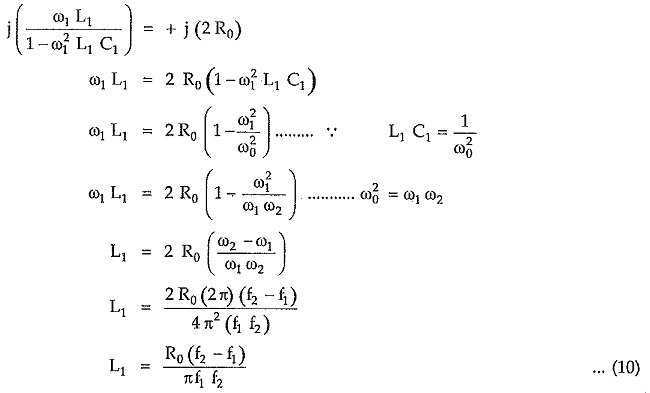For band elimination filter constant K section, frequency of resonance in series arms is given by,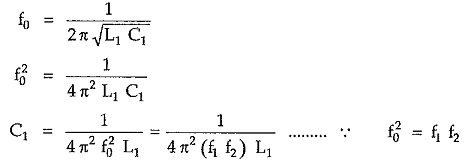Substituting value of L1 in above equation,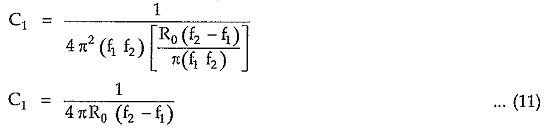From equation (6) we can write,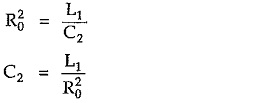Substituting value of L1 from equation (10),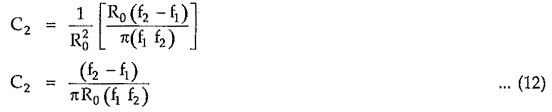Similarly from equation (6) we can write,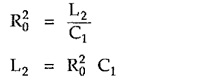Substituting value of C1 from equation (11),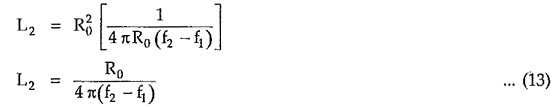Equations (10) to (13) are called design equations of prototype band elimination filter sections.

Scroll to Top